# Acceleration methods

Jump to: navigation, search

The theory and techniques for increasing the speed of convergence in the iterative solution of linear systems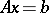. See Acceleration of convergence and the references given there.

The fundamental problem of linear algebra is the solution of finite-dimensional linear algebraic systems(a1)

in whichis a given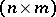-matrix. For simplicity, letbe an-matrix with real entries. The numerical solution of very large systems (e.g.,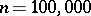) is not uncommon today (1996). Commonly, the saving grace of such large systems is their sparseness: most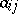are zero (cf. Sparse matrix). The classical direct method of solution, Gauss elimination, has at least three disadvantages: the pivoting operation fills in zeros, the operation count is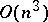, and the storage requirements when building a classical triangular factorization ofincreases superlinearly with.

For these reasons, indirect iterative methods for the solution of (a1) have recently come to the fore. Iterative methods may be roughly broken into two classes: splitting methods and gradient methods. Splitting methods decomposeaccording to(a2)

whereis non-singular (cf. Non-singular matrix). The solution to (a1) is then sought as the fixed point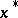of the equation(a3)

Accordingly, one picks an initial guess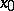and forms the iterates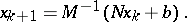(a4)

Provided that the spectral radius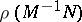is less than one, the iteration (a4) converges to the solutionof (a1) for any initial guess.

Gradient methods, on the other hand, form a variational problem from (a1). For example, minimizing the quadratic form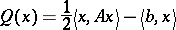(a5)

fora symmetric positive-definite matrix, is equivalent to the problem (a1).

Just as for the classical direct methods of solution of (a1), the indirect iterative methods in their original formulations are too slow for ever larger matrices. Therefore the original Jacobi iterative method (cf. Jacobi method)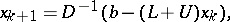(a6)

where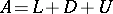has been written in terms of its lower-triangular, diagonal, and upper-triangular parts, has been accelerated by the Gauss–Seidel method(a7)

which in turn has been accelerated by the successive overrelaxation method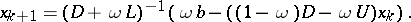(a8)

(Cf. also Hyperrelaxation method.) In (a8),is a free parameter which may be sought optimally. Generally, the error of a splitting iterative method is reduced by each iteration by a factor bounded by.

In like vein, the original gradient iterative method, steepest descent, in which the-th residual error vector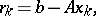(a9)

being the negative of the gradient of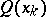hence defines the direction of steepest descent, converges more slowly as one nears the bottom of the quadratic surface (cf. also Steepest descent, method of). The convergence also becomes slow when the condition number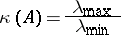(a10)

of the matrixis large. Hereanddenote the largest and smallest eigenvalues offorsymmetric positive-definite, and may be replaced in (a10) by their absolute values or, more generally, byandfor general matrices. Therefore, the steepest descent method has been accelerated by the conjugate-gradient method. Both steepest descent and conjugate gradient may be written as iterations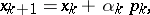(a11)

where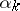denotes an optimal magnitude of change in the direction. In steepest descent,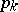is the residual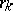of (a9) andis then chosen to minimize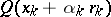. In conjugate gradient one takes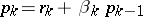, whereis chosen so thatis conjugate to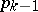, i.e.,(a12)

Thenis chosen to minimizeas before. Because the conjugate-gradient method may be seen to choose a new dimension each iteration, in theory (i.e., in the absence of roundoff errors) it must converge insteps. In practice it usually converges even faster than that.

The above acceleration methods, i.e., those based upon splittings and those based upon gradients, respectively, can be referred to as the classical acceleration methods: relaxation methods and direction methods, respectively. Recently (1996) these methods have been sharpened in a number of ways. Two of the most important sharpenings are Chebyshev acceleration and preconditioning acceleration. Both methods often involve polynomial iterations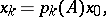(a13)

whereis some polynomial chosen from some knowledge or estimation of the eigenvalues of. These methods therefore make use of results and techniques from both approximation theory and spectral theory.

Chebyshev methods depend upon the property of the Chebyshev polynomials of minimizing the maximum error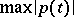forin some interval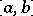. For example, for the conjugate-gradient method it may be shown that (see [a5])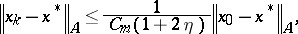(a14)

whereis the Chebyshev polynomial of the first kind of degree, where, and where. A disadvantage of Chebyshev methods in practice is their dependence upon knowledge or good estimates of the eigenvalues of.

Preconditioning has recently become the most important acceleration method. There are several preconditioning strategies, but the basic idea is to left or right multiplyin (a1) by some preconditioning matrix so that the transformed system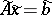is easier to solve. For example, ifapproximatesin some correct sense of representing's main invertibility features, i.e., so that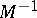approximates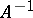in some good way, then (a1) is transformed by right preconditioning to the equivalent system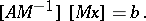(a15)

Left preconditioning yields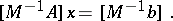(a16)

Two-sided preconditioning by multiplicatively splittinginto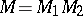becomes(a17)

Because the convergence rate of iterative methods generally depends on the condition numberof (a10), a rule of thumb has been to seek preconditionerssuch that the transformed systemhas lower, hopefully much lower, condition number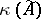.

There are roughly three generally successful theories for finding preconditioners: incomplete factorizations, Frobenius-norm methods and relaxation methods. The incomplete factorizations approximateby its approximate factorization into lower and upper triangular factors, but insisting on sparse approximations by dropping small elements. Then a conjugate-gradient method or Krylov-space variant of it (e.g., schemes such as ORTHOMIN or GMRES) may converge well. The Frobenius-norm methods, roughly, seek a weight matrix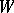such that the trace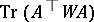is minimized. Often, an incomplete Cholesky factorization enters into applications of this method. Relaxation methods have been described above. Often, these are combined (see [a1]) with block Schur complement decompositions of. Relaxation methods such as (a6), (a7), (a8) are used today chiefly as preconditioners. Even the lowly Jacobi method (a6) can be a practical preconditioner on highly parallel computers when appropriately implemented.

Acceleration methods are also used for the iterative solution of the eigenvalue-eigenvector problem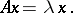(a18)

(a18) may be regarded as a special case of (a1) in whichand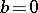. However, one must approximate the eigenvalueas one is approximating the eigenvector. Both Chebyshev and preconditioning acceleration methods enter into these (e.g., Arnoldi and Krylov) methods for eigenvalue-eigenvector computation. An important preconditioning method somewhat special to these problems is the so-called shift and invert method, using inverted shifts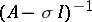to more widely disperse the eigenvalues (see [a4]).

There are other useful variations on preconditioning for accelerating convergence. Conjugate gradient can be applied as a preconditioner for any of the relaxation schemes. Other methods, such as multigrid schemes, have been successfully used as preconditioners (see [a3]). Preconditioning strategies can be cast in terms of a new theory of anti-eigenvaluesrather than the eigenvalue condition number(see [a2] and Anti-eigenvalue). Finding optimal preconditioners for an important specific application often usefully involves specific physical details of that application. For this reason, in practice preconditioning is sometimes labeled "black magic" .